# Math

Subcategories for the Math are listed below to provide more narrow searching within the Math category.

## Subcategories

 1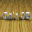Calc 3D Pro A collection of mathematical tools for highschool and university. 2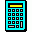RPN Engineering Calculator 240 solutions, 6 unique keypads: Standard, Engineering, Binary, Magnetics, Trigonometry, and Convert. 3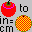32bit Convert It Converts any unit of measurement to another unit of measurement. 4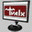Scientific Calculator Precision 54 Scientific calculator for scientists, engineers, teachers, and students. 5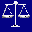APSW Instant Converter A simple, user friendly unit conversion program.